Here is a Cuemath rocket getting launched. It can go as high as 1000 ft.

After the engine is ignited, the rocket will go up for a few seconds.As the engine burns out, the rocket will be in freefall.

Now, Cooper will investigate the time and height attained by the rocket.He describes the path by a quadratic expression.

The word "quadratic" is derived from the word "quad" which means square.

In other words, a quadratic expression is a “polynomial of degree 2”.

In this mini-lesson, we will explore the world of quadratic expressions. You will get to learn how to solve quadratic expressions using the discriminant, simplifying quadratic expressions, factorizing expressions, quadratic expressions graphs, and other interesting facts around the topic. You can also check out the playful calculators to know more about the lesson and try your hand at solving a few interesting practice questions at the end of the page.

## Lesson Plan

 1 What Are Quadratic Expressions? 2 Important Notes on Quadratic Expressions 3 Solved Examples on Quadratic Expressions 4 Challenging Question on Quadratic Expressions 5 Interactive Questions on Quadratic Expressions

### Definition

An expression of the form $$ax^2+bx+c$$, where $$a \neq 0$$ is called a quadratic expression.

The standard form of a quadratic expression looks like this: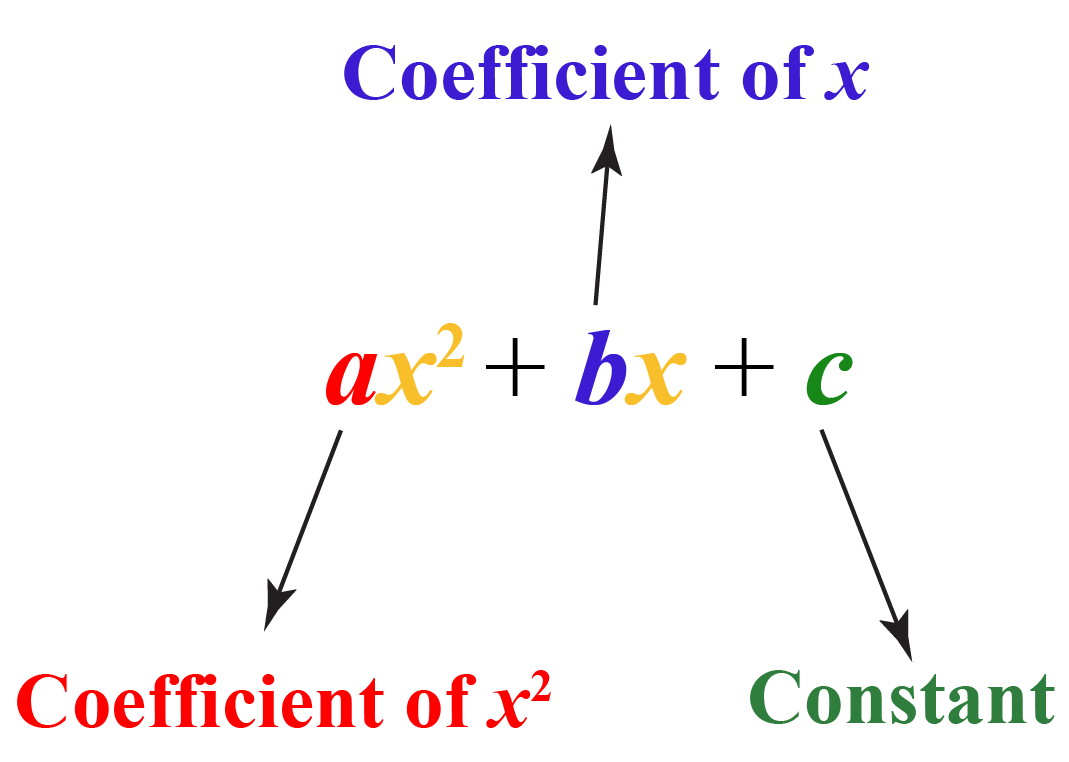Here are some examples of expressions:

Expressions Values of $$a$$, $$b$$, and $$c$$
1. $$8x^2+7x-1$$ \begin{align}a&=8 \\b&=7 \\c&=-1 \end{align}
2. $$4x^2-9$$ \begin{align}a&=4 \\b&=0 \\c&=-9 \end{align}
3. $$x^2+x$$ \begin{align}a&=1 \\b&=1 \\c&=0 \end{align}
4. $$6x-8$$ \begin{align}a&=0 \\b&=6 \\c&=-8 \end{align}

Look at Example 4

Here, $$a = 0$$. Therefore, it is not a quadratic expression. In fact, this is a linear expression.

The remaining expressions are quadratic expression examples.

## How Do You Identify a Quadratic Expression?

You are now aware of the standard form of a quadratic expression.

The quadratic expression in variable $$x$$ is $$ax^2+bx+c$$.

But always remember that $$a$$ is a non-zero value.

Sometimes, a quadratic expression is NOT written in its standard form.

Here are some quadratic expression examples:

 Expressions Steps to Follow Standard Form $$(x-1)(x+2)$$ Multiply the factors $$(x-1)$$ and $$(x+2)$$ $$x^2+x-2$$ $$-x^2+3x-1$$ Move expression by -1 $$x^2-3x+1$$ $$5x(x+3)-12x$$ Expand the term $$5x(x+3)$$ $$5x^2+3x$$ $$x^3-x(x^2+x-3)$$ Expand the term $$x(x^2+x-3)$$ and simplify $$x^2-3x$$

This is how the quadratic expression is represented on a graph.

This curve is called a parabola.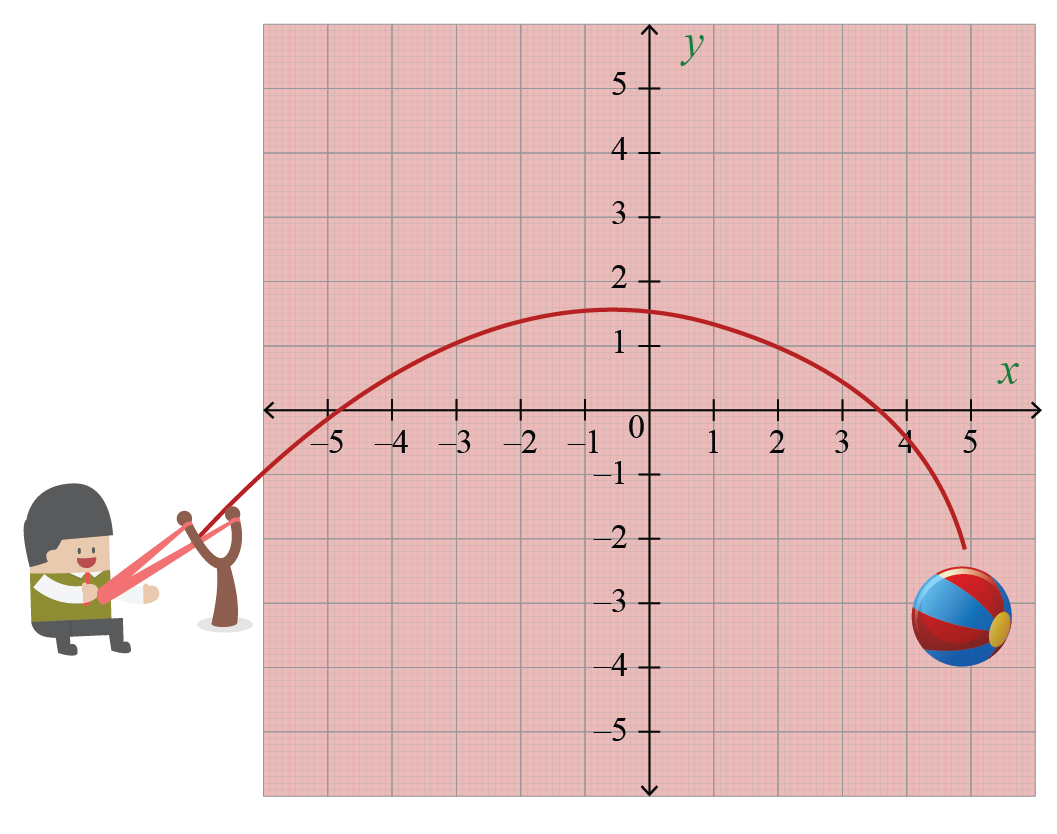Now let’s explore some quadratic expressions graphs using the simulation below.

Drag the values of $$a$$ to check for the variations of the upward parabola and downward parabola.

Dragging the values of $$b$$ will move the curve either right or left.

Dragging the values of constant $$c$$ will move the curve either up or down.

## Factorizing Expressions

We’ll do a few examples of factorizing expressions.

Example 1:

$4x-12x^2$

Given any quadratic expression, first, check for common factors.

In this example, check for the common factors among $$4x$$ and $$12x^2$$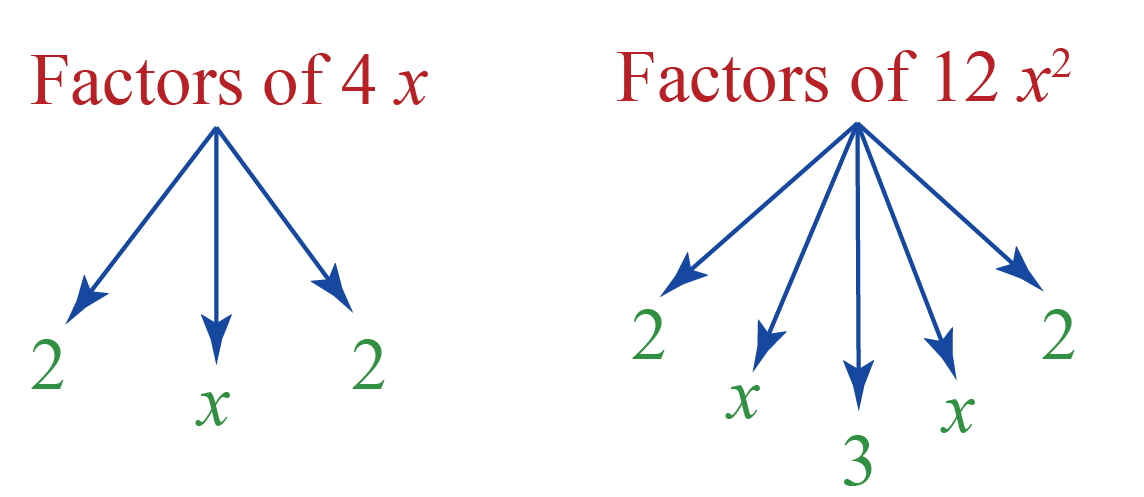We can observe that $$4x$$ is a common factor. Let’s take that common factor from the quadratic expression.

\begin{align}4x-12x^2&=4x(1-3x)\end{align}

Thus, by simplifying the quadratic expression, we get the expression

$4x-12x^2$

Example 2:

$x^2+5x+6$

We’ll factor this in two binomials, namely $$(x+a)$$ and $$(x+b)$$.

Expand $$(x+a)(x+b)$$.

\begin{align}(x+a)(x+b)&=x(x+b)+a(x+b)\\&=x^2+bx+ax+ab\\&=x^2+(a+b)x+ab\end{align}

Let’s try to find $$a$$ and $$b$$ such that $$(a+b)$$ is mapped to 5 and $$ab$$ is mapped to 6.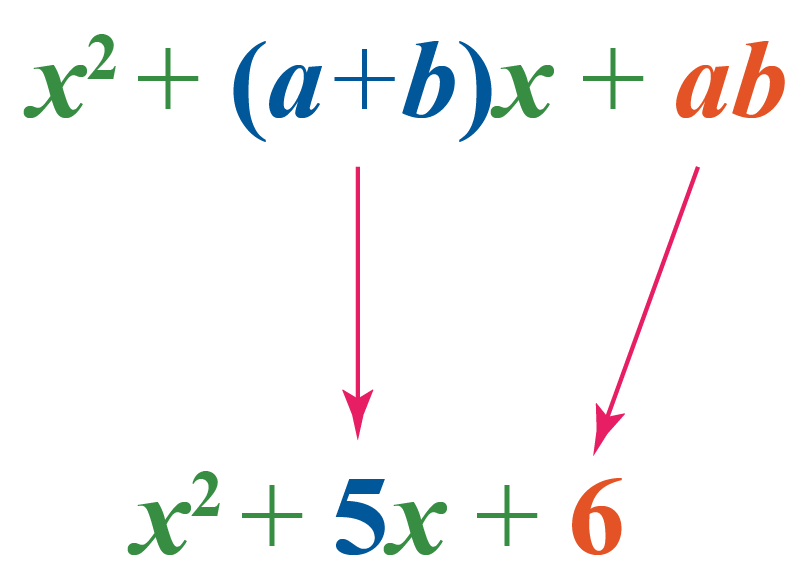The factors of $$6$$ are $$1$$, $$2$$, $$3$$, and $$6$$. Find the pairs $$a$$ and $$b$$ from $$1$$, $$2$$, $$3$$, and $$6$$ such that $$a+b=5$$ and $$ab=6$$

This is the right pair: $$2$$ and $$3$$, because, $$2+3 = 5$$ and $$2 \times 3=6$$

Thus, simplifying the quadratic expression we get

$(x+2)(x+3)$

## How Do You Solve Quadratic Expressions?

Sometimes working with a quadratic expression is difficult. But don’t worry! The quadratic formula is here to help.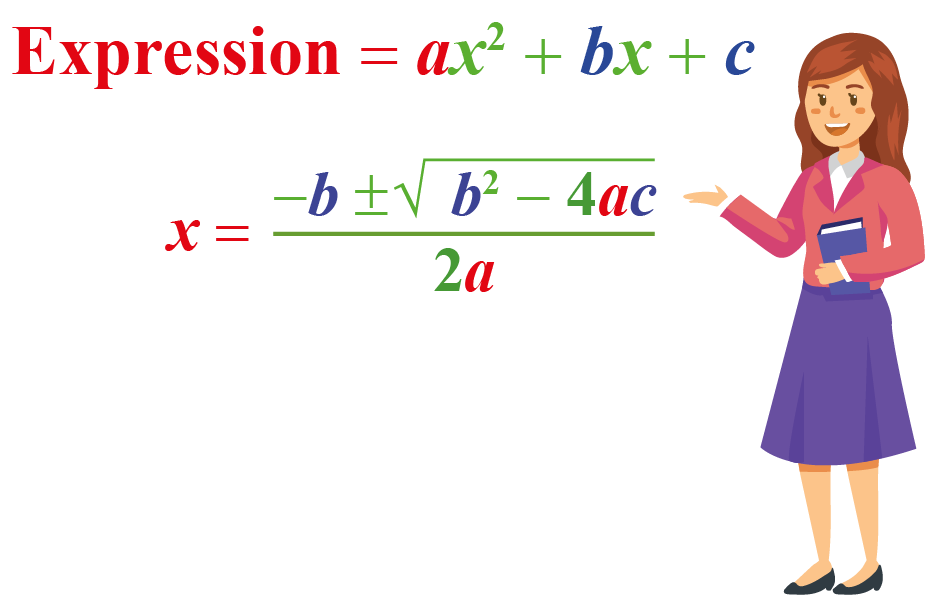This is also called a quadratic formula.

### Discriminant

Do you observe the term $$b^2-4ac$$ in the quadratic formula?

This is called a "discriminant" because it discriminates the zeroes of the quadratic expression based on its sign.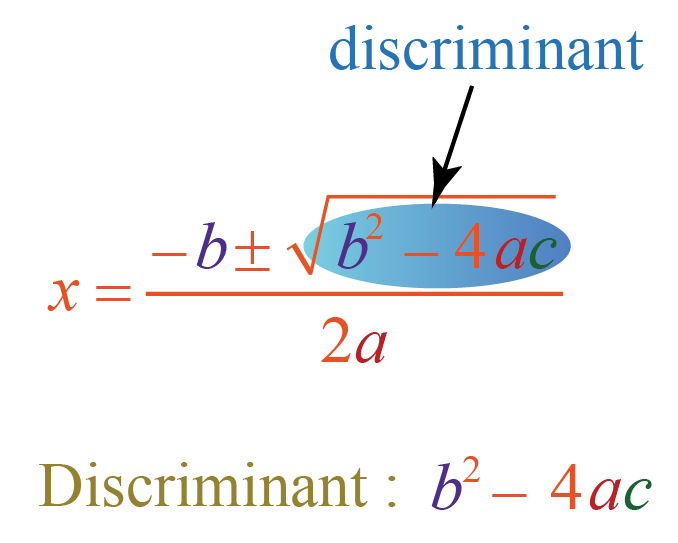To find the nature of zeroes of a quadratic expression, a discriminant is used.

• $$b^2-4ac>0$$

In this case, the quadratic expression has two distinct real zeroes.

• $$b^2-4ac=0$$

In this case, the quadratic expression has one repeated real zeroes.

• $$b^2-4ac<0$$

In this case, the quadratic expression has no real zeroes.

## Solved Examples

 Example 1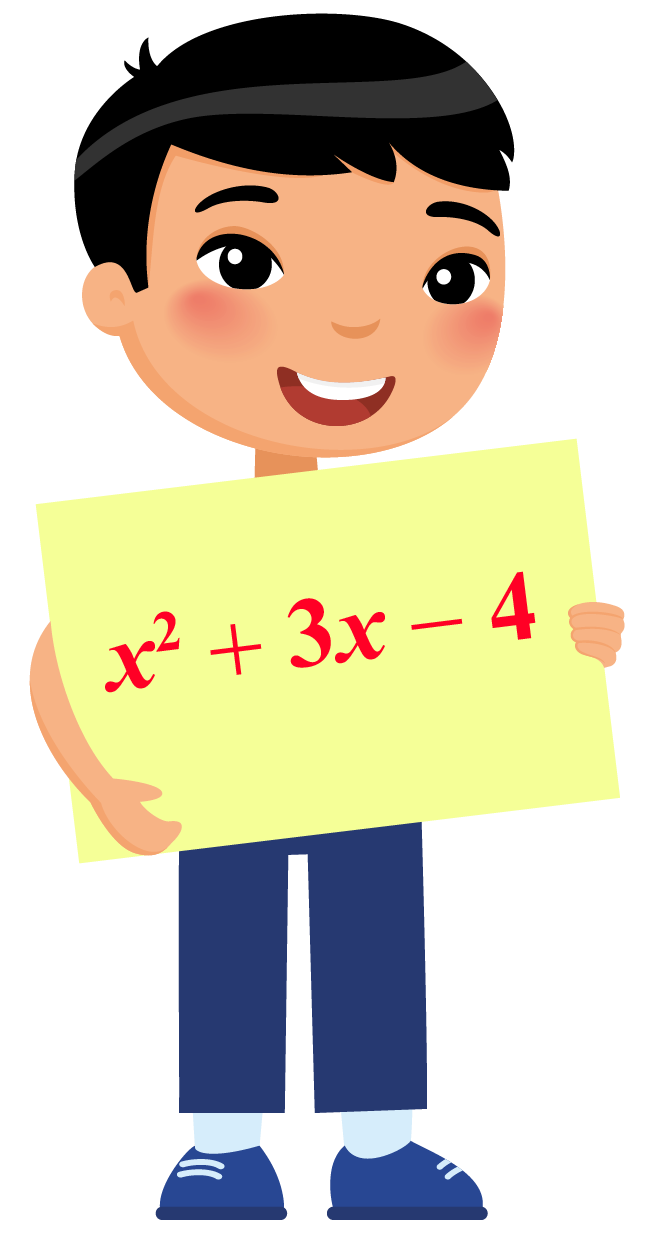Can you find the value of expression at $$x=-2$$?

Solution

Suppose we have an expression $$p(x)$$ in variable $$x$$.

The value of expression at $$k$$ is given by $$p(k)$$.

To find the value of expression at $$x=-2$$, substitute -2 for $$x$$ in the given expression.

\begin{align}(-2)^2+3(-2)-4&=4-6-4\\&=-6\end{align}

 $$\therefore$$, The value of expression at $$x=-2$$ is -6
 Example 2

Elsie has a two-digit secret lucky number with her.

She gives a few hints to her friend Mia to crack it.She says "It is the value of discriminant of the quadratic expression $$x^2+9x+14$$."

Can you guess the lucky number?

Solution

The quadratic expression is $$x^2+9x+14$$.

On comparing it with $$ax^2+bx+c$$, we get $$a=1$$, $$b=9$$, and the constant $$c=14$$.

Let's use the discriminant formula to find the discriminant.

\begin{align}\text{Discriminant}&=b^2-4ac\\&=9^{2}-4(1)(14)\\&=81-56\\&=25\end{align}

 $$\therefore$$ The lucky number is 25
 Example 3

Flora loves collecting flowers.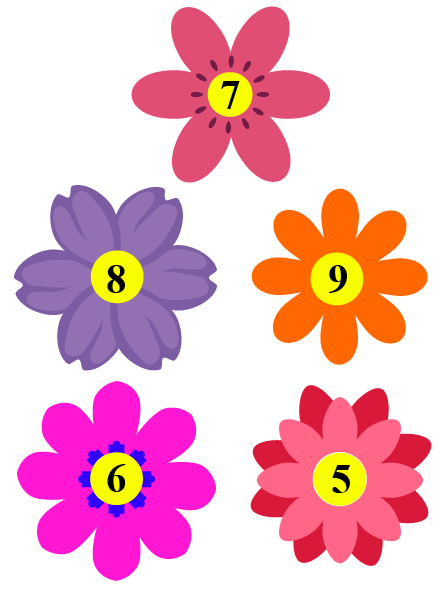She wants to choose a number such that it is the solution to the quadratic expression $$x^{2}-8x+12$$.

Can you help her choose the correct number?

Solution

Compare the quadratic expression $$x^{2}-8x+12$$ with the standard form of quadratic expression $$ax^{2}+bx+c$$.

We get, $$a=1$$, $$b=-8$$ and the constant $$c=12$$

Now calculate the solution of the quadratic expression by using the quadratic formula.

\begin{aligned}x&=\frac{-b\pm\sqrt{b^2-4ac}}{2a}\\&=\frac{-(-8)\pm\sqrt{(-8)^2-4(1)(12)}}{2}\\&=\frac{8\pm\sqrt{64-48}}{2}\\&=\frac{8\pm\sqrt{16}}{2}\\&=\frac{8\pm4}{2}\\x&=6,2\end{aligned}

The number 2 is not on the flowers. Therefore, she will choose the flower on which number 6 is written.

 $$\therefore$$ The correct number is 6Challenging Questions

Riley is trying to find a quadratic expression. The conditions for the quadratic expression are given as:

• The expression is free from the term having a linear degree of $$x$$.
• The solutions of the quadratic expression are 3 and -3

Can you help her to find the term independent of $$x$$ in the expansion of quadratic expression and the quadratic expression?

## Interactive Questions

Here are a few activities for you to practice.

## Let's Summarize

The mini-lesson targeted the fascinating concept of quadratic expression. The math journey around quadratic expression starts with what a student already knows, and goes on to creatively crafting a fresh concept in the young minds. Done in a way that is not only relatable and easy to grasp but will also stay with them forever. Here lies the magic with Cuemath.

At Cuemath, our team of math experts is dedicated to making learning fun for our favorite readers, the students!

Through an interactive and engaging learning-teaching-learning approach, the teachers explore all angles of a topic.

Be it worksheets, online classes, doubt sessions, or any other form of relation, it’s the logical thinking and smart learning approach that we, at Cuemath, believe in.

### 1. What is a quadratic expression in math?

An expression of the form $$ax^2+bx+c$$, where $$a \neq 0$$ is called a quadratic expression in variable $$x$$.

### 2. What are the 3 forms of a quadratic equation?

The three forms of a quadratic equation are

1. Standard form
2. Factored form
3. Vertex form

### 3. What does it mean to factor a quadratic expression?

The factored form of a quadratic expression is the multiplication of two linear expressions.

### 4. Which is a quadratic function?

A function of the form $$f(x)=ax^2+bx+c$$, where $$a \neq 0$$ is called a quadratic function in variable $$x$$.

### 5. What does quadratic mean?

The word "quadratic" is derived from the word "quad" which means square.

### 6. How do you know if a polynomial is quadratic?

If the coefficient of $$x^2$$ is non-zero, then the expression is quadratic.

### 7. What are the characteristics of a quadratic expression?

The characteristics of a quadratic expression are:

1. The standard form of the quadratic expression is $$ax^2+bx+c$$ where $$a\neq0$$.
2. A quadratic expression has at most two zeroes.
3. The curve of the quadratic expression is in the form of a parabola.

### 8. What are the terms in an expression?

An expression can have only a constant or an addition of constant and variable terms having different degrees.

### 9. How do you write quadratic equations in standard form?

The standard form of a quadratic equation is $$ax^2+bx+c=0$$, where $$a \neq 0$$ in variable $$x$$..

### 10. How do you simplify quadratic equations?

Quadratic equations can be simplified by factorization.

More Important Topics
Numbers
Algebra
Geometry
Measurement
Money
Data
Trigonometry
Calculus
More Important Topics
Numbers
Algebra
Geometry
Measurement
Money
Data
Trigonometry
Calculus